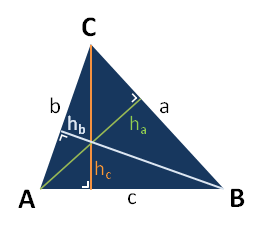# Triangle formulas

List of triangle formulas used to calculate angles, sides, height, area and perimter of a triangle.#### Law of sines

 a = b = c sin A sin B sin C

The law of sines can be used to calculate the remaining sides of a triangle when two angles and a side are known or two sides and one of the non-enclosed angles are known.

### c2 = a2 + b2 - 2ab cos(angle C)

The law of cosines can be used to calculate the third side of a triangle when two sides and their enclosed angle are known or if all three sides are known.

### a2 + b2 = c2

The Pythagorean theorem can only be used with right-angled triangles: if the lengths of any two sides are known the length of the third side can be calculated.

### angle A + angle B + angle C = 180o

If two angles are know, the third angle can be calculated.